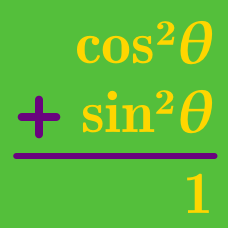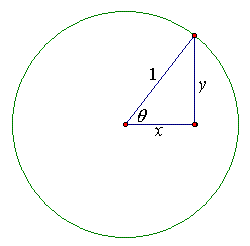Geometry

Pythagorean Identities Warmup

Given $\sin^2(\theta) + \cos^2(\theta) = 1$, which of the following is true?

True or False: $\sin^2(\theta) - \cos^2(\theta) + 1 = 2\sin^2(\theta)$.

(Hint: Use the identity $\sin^2(\theta) + \cos^2(\theta) = 1$.)

Which is these is equivalent to $\cos^2(\theta)\sec^2(\theta) - \cos^2(\theta),$ over values of $\theta$ for which the given expression is defined?Which of these is equivalent to $x$?

If $\sin^2(\theta) = \frac{9}{25}$, what is $\cos^2(\theta) ?$

×